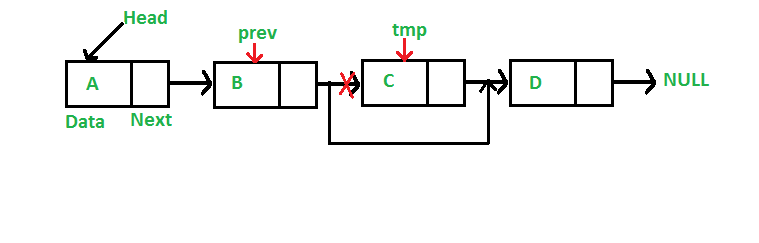# Python Program for Deleting a Node in a Linked List

• Last Updated : 18 May, 2022

We have discussed Linked List Introduction and Linked List Insertion in previous posts on a singly linked list.
Let us formulate the problem statement to understand the deletion process. Given a ‘key’, delete the first occurrence of this key in the linked list

Iterative Method:
To delete a node from the linked list, we need to do the following steps.
1) Find the previous node of the node to be deleted.
2) Change the next of the previous node.
3) Free memory for the node to be deleted.Since every node of the linked list is dynamically allocated using malloc() in C, we need to call free() for freeing memory allocated for the node to be deleted.

## Python3

 `# A complete working Python3 program to``# demonstrate deletion in singly ``# linked list with class `` ` `# Node class ``class` `Node: `` ` `    ``# Constructor to initialize the node object ``    ``def` `__init__(``self``, data): ``        ``self``.data ``=` `data ``        ``self``.``next` `=` `None`` ` `class` `LinkedList: `` ` `    ``# Function to initialize head ``    ``def` `__init__(``self``): ``        ``self``.head ``=` `None`` ` `    ``# Function to insert a new node at the beginning ``    ``def` `push(``self``, new_data): ``        ``new_node ``=` `Node(new_data) ``        ``new_node.``next` `=` `self``.head ``        ``self``.head ``=` `new_node `` ` `    ``# Given a reference to the head of a list and a key, ``    ``# delete the first occurrence of key in linked list ``    ``def` `deleteNode(``self``, key): ``         ` `        ``# Store head node ``        ``temp ``=` `self``.head `` ` `        ``# If head node itself holds the key to be deleted ``        ``if` `(temp ``is` `not` `None``): ``            ``if` `(temp.data ``=``=` `key): ``                ``self``.head ``=` `temp.``next``                ``temp ``=` `None``                ``return`` ` `        ``# Search for the key to be deleted, keep track of the ``        ``# previous node as we need to change 'prev.next' ``        ``while``(temp ``is` `not` `None``): ``            ``if` `temp.data ``=``=` `key: ``                ``break``            ``prev ``=` `temp ``            ``temp ``=` `temp.``next`` ` `        ``# if key was not present in linked list ``        ``if``(temp ``=``=` `None``): ``            ``return`` ` `        ``# Unlink the node from linked list ``        ``prev.``next` `=` `temp.``next`` ` `        ``temp ``=` `None`` ` ` ` `    ``# Utility function to print the linked LinkedList ``    ``def` `printList(``self``): ``        ``temp ``=` `self``.head ``        ``while``(temp): ``            ``print` `(``" %d"` `%``(temp.data)), ``            ``temp ``=` `temp.``next`` ` ` ` `# Driver program ``llist ``=` `LinkedList() ``llist.push(``7``) ``llist.push(``1``) ``llist.push(``3``) ``llist.push(``2``) `` ` `print` `(``"Created Linked List: "``)``llist.printList() ``llist.deleteNode(``1``) ``print` `("``Linked ``List` `after Deletion of ``1``:")``llist.printList() `` ` `# This code is contributed by Nikhil Kumar Singh (nickzuck_007) `

Output:
```Created Linked List:
2  3  1  7
Linked List after Deletion of 1:
2  3  7```

Please refer complete article on Linked List | Set 3 (Deleting a node) for more details!

My Personal Notes arrow_drop_up